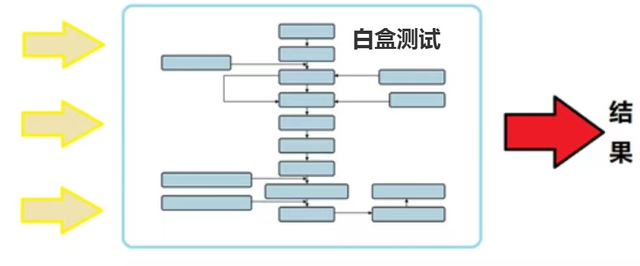# 白盒测试的方法规律和逻辑路径覆盖法的六大内容实例讲解！

"白盒"法全面了解程序内部逻辑结构、对所有逻辑路径进行测试。"白盒"法是穷举路径测试。在使用这一方案时，测试者必须检查程序的内部结构，从检查程序的逻辑着手，得出测试数据。贯穿程序的独立路径数是天文数字。但即使每条路径都测试了仍然可能有错误。1.语句覆盖每条语句至少执行一次。

2.判定覆盖每个判定的每个分支至少执行一次。

3.条件覆盖每个判定的每个条件应取到各种可能的值。

4.判定/条件覆盖同时满足判定覆盖条件覆盖。

5.条件组合覆盖每个判定中各条件的每一种组合至少出现一次。

6.路径覆盖使程序中每一条可能的路径至少执行一次。

7.六种覆盖标准发现错误的能力呈由弱至强的变化。

1.语句覆盖 Statement coverage    2.判定覆盖 Decision coverage

3.条件覆盖 Condition coverage    4.条件/判定覆盖 Condition/decision coverage

5.条件组合覆盖 Condition combination coverage   6.路径覆盖 Path coverage

if (a>1) and (b = 0)

then x = x / a

if (a = 2) or (x > 1)

then x = x+ 1

1、根据以上程序段画出程序流程图

2、按照要求给出六种逻辑覆盖的测试用例

#### 1.语句覆盖

-》定义：要求设计足够多的测试用例，使得程序中每条语句至少被执行一次

-》缺点：语句覆盖常常被人指责为“最弱的覆盖”，它只管覆盖代码中的执行语句，却不考虑各种分支的组合

-》题解分析：

1、可执行语句4条、变量3个(a,b,x)

2、设计最少的案例，每个语句至少执行一次

-》题解:

a=2, b=0, x=4   覆盖路径：a-c-e

(还有其他测试用例方案，满足条件即可)

(路径b、d没有被覆盖，这是其缺点所在)

#### 2.判定覆盖

(所有分支路径都至少经过1次)

-》题解分析：需要覆盖的路径为：(a-c-e、a-b-d) 或 (a-b-e、a-c-d)

“判定覆盖”比“语句覆盖”严格，因为如果每个分支都执行过了，则每个语句也就执行过了。但是，“判定覆盖”还是很不够的，比如选择a-b-e和a-c-d案例就没有覆盖到a-d-b的情况

-》题解：测试用例如下：

a = 2, b = 0, x = 4 覆盖路径：a-c-e

a = 0, b = 0, x = 1 覆盖路径：a-b-d

a = 2, b =2, x = 2 覆盖路径：a-b-e

a = 3, b = 0, x = 3 覆盖路径：a-c-d

#### 3.条件覆盖

-》定义：选择足够多的测试用例，使程序中每个判定的每个条件取得各种可能的结果

-》题解分析：

-》题解：

a = 2, b = 0, x = 4 覆盖(a > 1, a = 2, b = 0, x > 1)

a = 1, b = 1, x = 1 覆盖(a <= 1, b != 0, x <= 1)

#### 4.判定-条件覆盖

-》定义：判定条件覆盖是设计足够的测试用例，得使判断中每个条件的所有可能取值至少执行一次，同时每个判断本身所有可能结果也至少执行一次。缺点是忽略了条件的组合情况

-》简单记忆：判定覆盖、条件覆盖 取交集

-》分析：

x>3andy=1

1.使得判定为真

2.使得判定为假

3.使得x>3

4.使得x<=3

5.使得y=1

6.使得y!=1

-》题解分析：

-》题解：

a = 2, b = 0, x = 4 覆盖路径a-c-e，覆盖条件(a > 1, a = 2, b = 0, x > 1)

a = 1, b = 1, x = 1 覆盖路径a-b-d，覆盖条件(a <= 1, b != 0, x <= 1)

#### 5.条件组合覆盖

-》定义：选择足够的测试用例，使得每个判定中条件的各种可能组合都至少出现一次、

-》分析：满足“条件组合覆盖”的测试用例是一定满足“判定覆盖”、“条件覆盖”和“判定/条件覆盖”

-》题解分析：

a > 1, b = 0

a>1, b != 0

a <= 1, b = 0

a <= 1, b != 0

a = 2, x > 1

a = 2, x< = 1

a != 2, x > 1

a != 2, x <= 1

-》题解：

a = 2, b = 0, x = 4 覆盖组合1，5

a = 2, b = 1, x = 1 覆盖组合2，6

a = 1, b = 0, x = 3 覆盖组合3，7

a = 1, b = 2, x = 1 覆盖组合4，8

#### 6.路径覆盖

-》定义：选取足够多的测试数据，使程序的每条可能路径都至少执行一次（如果程序图中有环，则要求每个环至少经过一次）

-》分析：覆盖程度最高的就是路径覆盖，因为其覆盖程序中所有可能的路径

-》题解分析：需要覆盖的路径为：a-c-e、 a-b-d、 a-b-e、 a-c-d

a = 2, b = 0, x = 4 覆盖路径：a-c-e

a = 1, b = 0, x = 1 覆盖路径：a-b-d

a = 2, b = 1, x = 1 覆盖路径：a-b-e

a = 3, b = 0, x = 4 覆盖路径：a-c-d